### Home > MC1 > Chapter 8 > Lesson 8.3.2 > Problem8-99

8-99.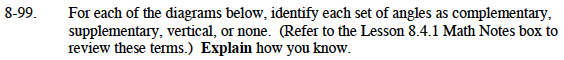See the excerpt from Lesson 8.4.1 Math Notes box below for help with this problem.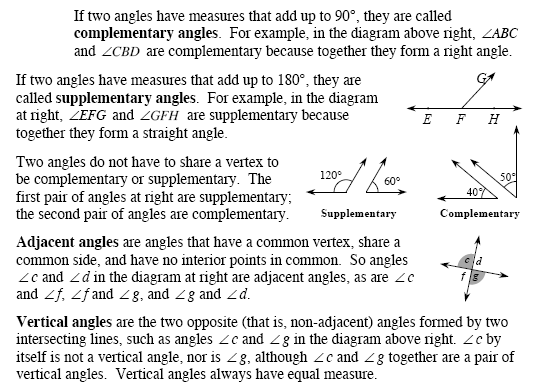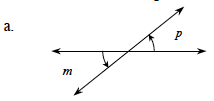Vertical angles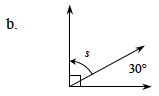What is the sum of s + 30°? Remember that a square indicates a right angle.

These are complementary angles.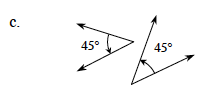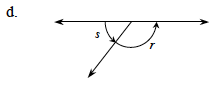For parts (c) and (d), think about what the sum of the two given angles is. Look at the definitions in the Math Notes if you need help.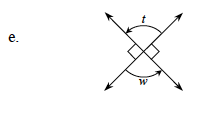Angles t and w are related in two ways. How are they positioned? What is their sum?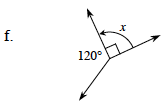Are x and 120° related in any special way?

None